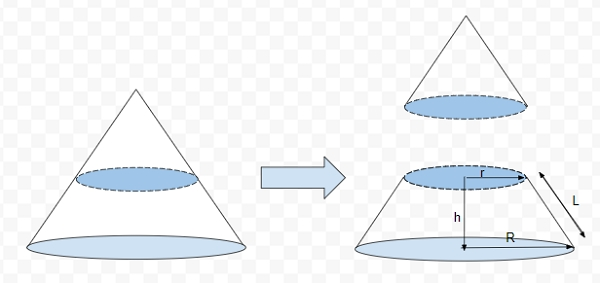# Program for Volume and Surface area of Frustum of Cone in C++

## What is Frustrum of cone?

Frustum of a cone is formed by cutting the tip of a cone leaving lower and upper base known as frustum as shown in the figure. The upper base of frustum will have radius ‘r’, lower base will have radius ‘R’ with height ‘h’ and slant height ‘L’

Given below is the figure of Frustrum of cone## Problem

Given with slant height, height, upper base radius ‘r’ and lower radius ‘R’, the task is to calculate the volume and surface area of Frustum of cone.

To calculate the volume and surface area of Frustum of cone there is a formula

Volume (V) = 1/3 * pi * h(r2 + R2 + r*R)
Curved Surface Area (CSA) = pi * l(R + r)
Total Surface Area (TSA) = pi * l(R + r) + pi(R2 + r2)

## Example

Input-: r=4 R=9 h=12 L=13
Output-: Volume Of Cone : 1671.33
Curved Surface Area Of Cone : 530.929
Total Surface Area Of Cone : 835.663

## Algorithm

Start
Step 1 -> define macro as
#define pi 3.14
Step 2 -> Declare function to calculate Volume of cone
float volume(float r, float R, float h)
return (float(1) / float(3)) * pi * h * (r * r + R * R + r * R)
Step 3 -> Declare function to calculate Curved Surface area of cone
float CSA(float r, float R, float l)
return pi * l * (R + r)
Step 4 -> Declare function to calculate Total Surface area of cone
float TSA(float r, float R, float l, float h)
return pi * l * (R + r) + pi * (r * r + R * R)
step 5 -> In main()
declare variables as R1=4, R2=9, slantHeight=13 and height=12
call volume(R1, R2, height)
Call CSA(R1, R2, slantHeight)
TSA(R1, R2,slantHeight, height)
Stop

## Example

Live Demo

#include <iostream>
#define pi 3.14159
using namespace std;
// Function to calculate Volume of cone
float volume(float r, float R, float h){
return (float(1) / float(3)) * pi * h * (r * r + R * R + r * R);
}
// Function to calculate Curved Surface area of cone
float CSA(float r, float R, float l){
return pi * l * (R + r);
}
// Function to calculate Total Surface area of cone
float TSA(float r, float R, float l, float h){
return pi * l * (R + r) + pi * (r * r + R * R);
}
int main(){
float R1 = 4;
float R2 = 9;
float slantHeight = 13;
float height = 12;
cout << "Volume Of Cone : "<< volume(R1, R2, height)<< endl;
cout << "Curved Surface Area Of Cone : "<<CSA(R1, R2, slantHeight)<< endl;
cout << "Total Surface Area Of Cone : "<<TSA(R1, R2,slantHeight, height);
return 0;
}

## Output

Volume Of Cone : 1671.33
Curved Surface Area Of Cone : 530.929
Total Surface Area Of Cone : 835.663